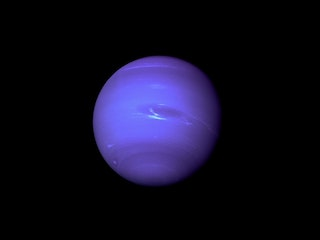# Work with numbers in lists

Completed

So far, you've been using planet names in a list. You might wonder about working with other data types, like numbers.

Did you know that gravity on other planets is stronger or weaker depending on the planet's mass or size? Gravity is often measured in G, where the gravity on earth is 1 and other planets are measured relative to Earth.

The gravity on the moon is 0.166 G, which is why astronauts can jump so high on the moon. The gravity on Neptune is 1.12 G, so jumping is harder. Even great athletes would struggle to jump over 2 meters on Neptune.## Store numbers in lists

To store numbers with decimal places in Python, you use the float type. To create a float, you enter the number with the decimal place and assign it to a variable:

gravity_on_earth = 1.0
gravity_on_the_moon = 0.166


The following code creates a list that shows the gravitational forces of all eight planets in the solar system, in G:

gravity_on_planets = [0.378, 0.907, 1, 0.377, 2.36, 0.916, 0.889, 1.12]


In this list, gravity_on_planets is the gravity on Mercury (0.378 G), gravity_on_planets is the gravity on Venus (0.907 G), and so on.

On Earth, a double-decker bus weighs 124,054 Newtons (N). On Mercury, where the gravity is 0.378 G, the same bus weighs 124,054 Newtons multiplied by 0.378. In Python, to multiply two values, you use the * symbol.

In the following example, you can work out the weight of a double-decker bus on different planets by getting the value from the list:

gravity_on_planets = [0.378, 0.907, 1, 0.377, 2.36, 0.916, 0.889, 1.12]
bus_weight = 124054 # in Newtons, on Earth

print("On Earth, a double-decker bus weighs", bus_weight, "N")
print("On Mercury, a double-decker bus weighs", bus_weight * gravity_on_planets, "N")

On Earth, a double-decker bus weighs 124054 N
On Mercury, a double-decker bus weighs 46892.4 N


## Use min() and max() with lists

Python has built-in functions for calculating the biggest and smallest numbers in a list. The max() function returns the largest number, and min() returns the smallest. So min(gravity_on_planets) returns the smallest number in the gravity_on_planets list, which is 0.377 (Mars).

The following code calculates the minimum and maximum weights in the solar system by using those functions:

gravity_on_planets = [0.378, 0.907, 1, 0.377, 2.36, 0.916, 0.889, 1.12]
bus_weight = 12650 # in Newtons, on Earth

print("On Earth, a double-decker bus weighs", bus_weight, "N")
print("The lightest a bus would be in the solar system is", bus_weight * min(gravity_on_planets), "N")
print("The heaviest a bus would be in the solar system is", bus_weight * max(gravity_on_planets), "N")

On Earth, a double-decker bus weighs 124054 N
The lightest a bus would be in the solar system is 46768.35 N
The heaviest a bus would be in the solar system is 292767.44 N